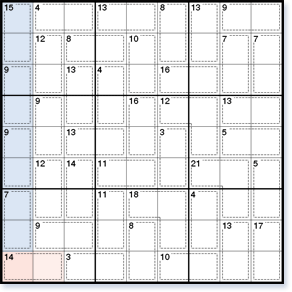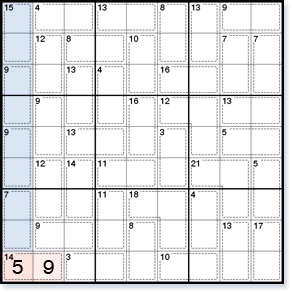# Simple Killer Sudoku solving techniques

Killer Sudoku adds a new dimension to standard Sudoku, requiring arithmetic to solve. You will need new specialised Killer Sudoku solving techniques to progress in these puzzles besides the standard Sudoku techniques you will already know.

## The 45 Rule

An essential Killer Sudoku solving technique is the "45 rule". This uses the fact that every row, column and block must contain each of the numbers 1 to 9 once. Therefore, the total of all numbers in one row, column or block will always be 45.Figure 1

Take the first column of the Killer Sudoku shown in Figure 1. The numbers in this column will add up to 45. Additionally, because the first four cages in this column (the 15 cage, the two 9 cages and the 7 cage) lie completely within the column, the numbers in the first eight squares total 15 + 9 + 9 + 7 = 40. Call this the 'inside total', because it is the total of all cages which lie completely inside the column. Now, as the whole column must add up to 45, the ninth square (the 'inside' square in this case as it is inside the column) must be 45 – 40 = 5. The 14 cage can now be completed by solving the other square as a 9.

This could also have been solved another way, by finding the 'outside' total - the total of all cages within the column, including the one lying partly outside. This is 15 + 9 + 9 + 7 + 14 = 54. Again, as all the squares within the column must add up to 45, the 'outside' square is 54 – 45 = 9. In the case, it was easier to calculate the inside total, but if the cage lying partly outside has one square outside and more than one square inside, the outside total should be used to calculate the outside square.Figure 2

In general, to use the 45 rule, look for a row, column or block where all cages except one lie completely inside.

For an inside square, the solution is always 45 – the inside total. For an outside square, the solution is always the outside total – 45.